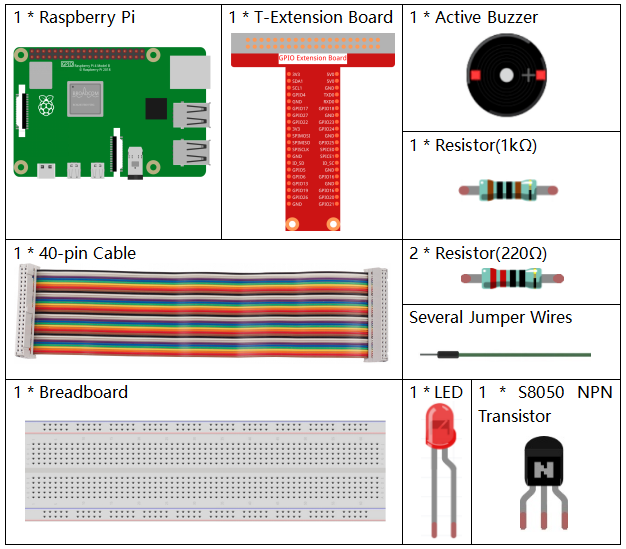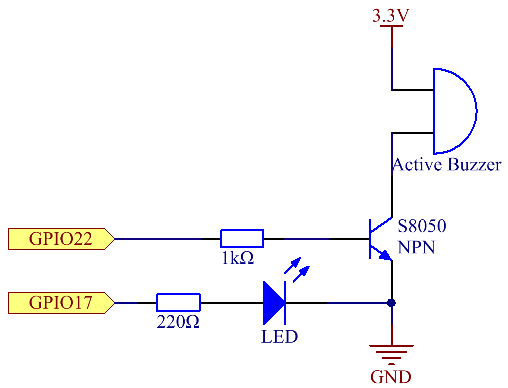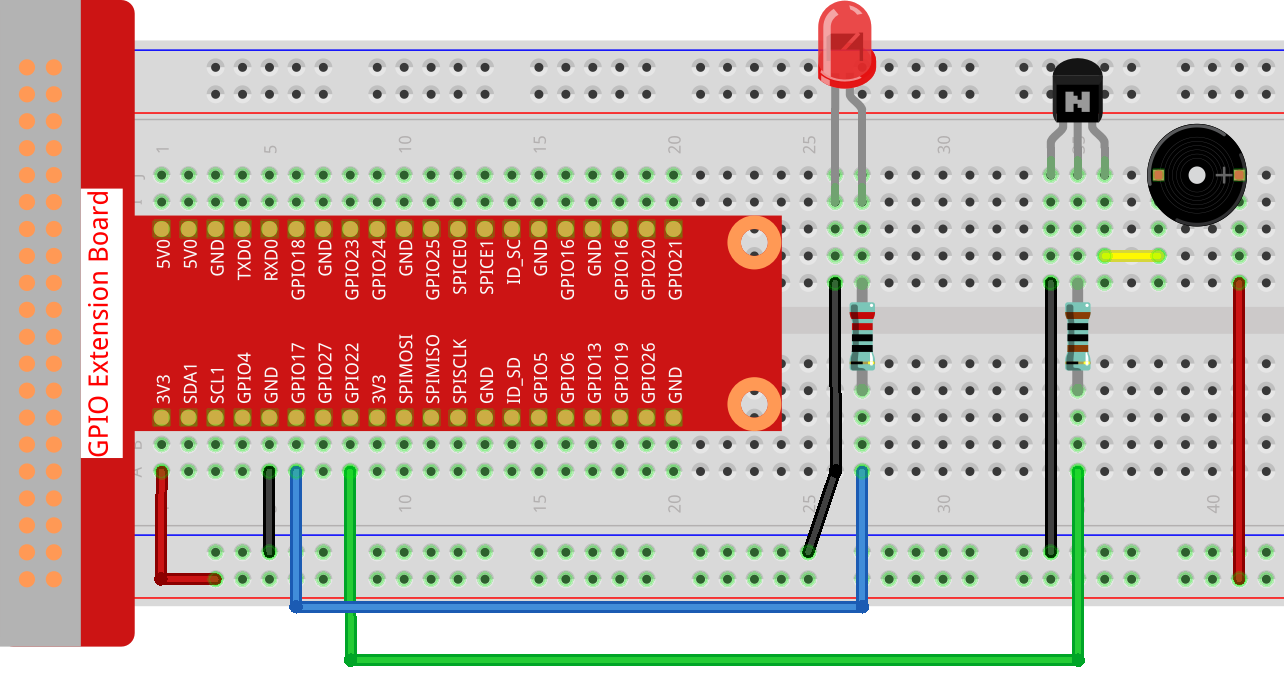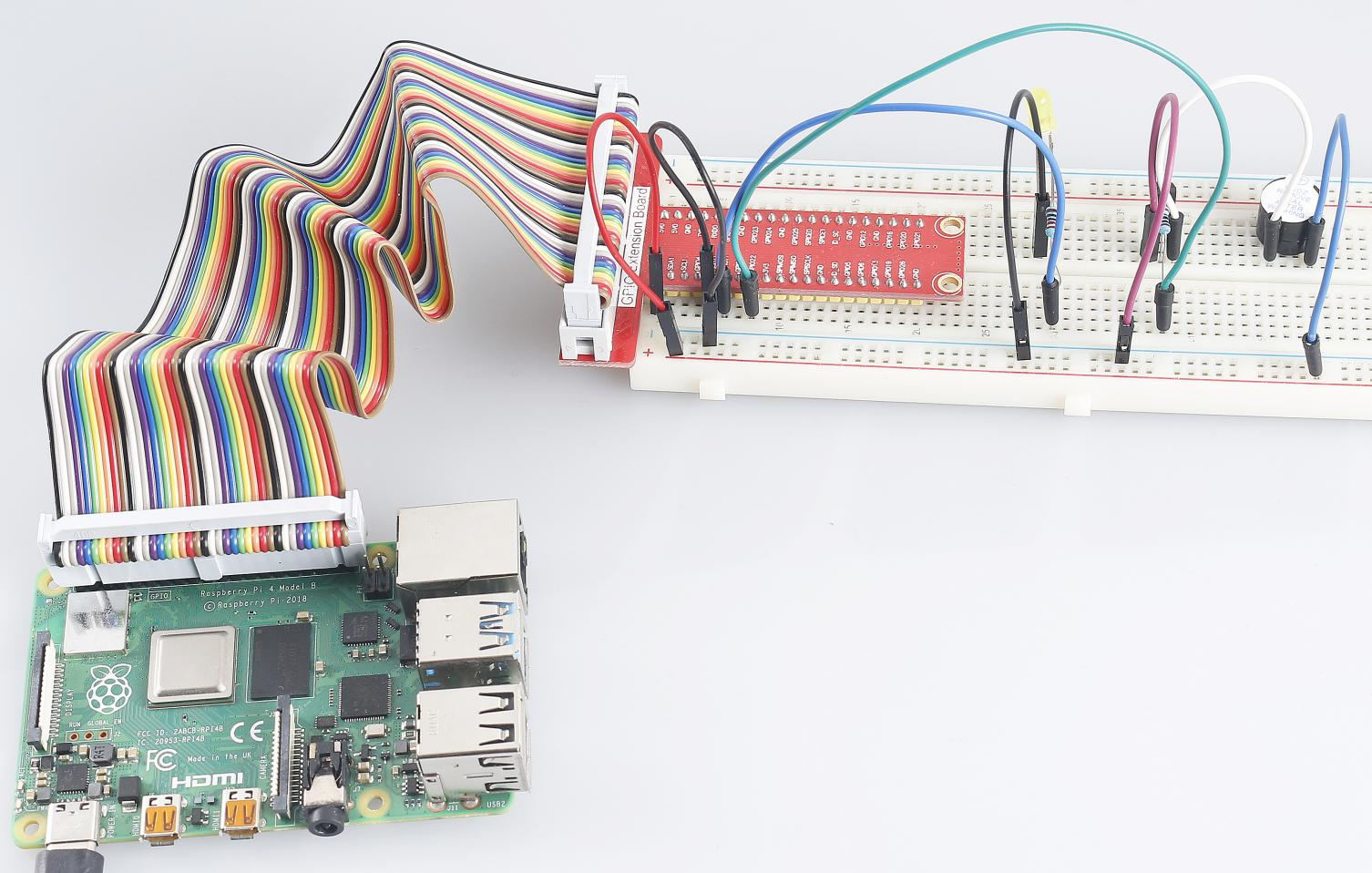# 3.1.10 Morse Code Generator¶

## Introduction¶

In this project, we’ll make a Morse code generator, where you type in a series of English letters in the Raspberry Pi to make it appear as Morse code.

## Required Components¶

In this project, we need the following components.Name

ITEMS IN THIS KIT

Raphael Kit

337

Raphael Kit

COMPONENT INTRODUCTION

GPIO Extension Board

Jumper Wires

Resistor

LED

Buzzer

-

Transistor

## Schematic Diagram¶

 T-Board Name physical wiringPi BCM GPIO17 Pin 11 0 17 GPIO22 Pin 15 3 22## Experimental Procedures¶

Step 1: Build the circuit. (Pay attention to poles of the buzzer: The one with + label is the positive pole and the other is the negative.)Step 2: Open the code file.

```cd ~/raphael-kit/c/3.1.10/
```

Step 3: Compile the code.

```gcc 3.1.10_MorseCodeGenerator.c -lwiringPi
```

Step 4: Run the executable file above.

```sudo ./a.out
```

After the program runs, type a series of characters, and the buzzer and the LED will send the corresponding Morse code signals.

Note

If it does not work after running, or there is an error prompt: "wiringPi.h: No such file or directory", please refer to Install and Check the WiringPi.

Code Explanation

```struct MORSE{
char word;
unsigned char *code;
};

struct MORSE morseDict[]=
{
{'A',"01"}, {'B',"1000"}, {'C',"1010"}, {'D',"100"}, {'E',"0"},
{'F',"0010"}, {'G',"110"}, {'H',"0000"}, {'I',"00"}, {'J',"0111"},
{'K',"101"}, {'L',"0100"}, {'M',"11"}, {'N',"10"}, {'O',"111"},
{'P',"0110"}, {'Q',"1101"}, {'R',"010"}, {'S',"000"}, {'T',"1"},
{'U',"001"}, {'V',"0001"}, {'W',"011"}, {'X',"1001"}, {'Y',"1011"},
{'Z',"1100"},{'1',"01111"}, {'2',"00111"}, {'3',"00011"}, {'4',"00001"},
{'5',"00000"},{'6',"10000"}, {'7',"11000"}, {'8',"11100"}, {'9',"11110"},
{'0',"11111"},{'?',"001100"}, {'/',"10010"}, {',',"110011"}, {'.',"010101"},
{';',"101010"},{'!',"101011"}, {'@',"011010"}, {':',"111000"}
};
```

This structure MORSE is the dictionary of the Morse code, containing characters A-Z, numbers 0-9 and marks “?” “/” “:” “,” “.” “;” “!” “@” .

```char *lookup(char key,struct MORSE *dict,int length)
{
for (int i=0;i<length;i++)
{
if(dict[i].word==key){
return dict[i].code;
}
}
}
```

The function `lookup()` works by “checking the dictionary”. Define a `key` search the same words as `key` in the structure `morseDict` and return the corresponding information— `code` of the certain word.

```void on(){
digitalWrite(ALedPin,HIGH);
digitalWrite(BeepPin,HIGH);
}
```

Create a function `on()` to start the buzzer and the LED.

```void off(){
digitalWrite(ALedPin,LOW);
digitalWrite(BeepPin,LOW);
}
```

The function `off()` turns off the buzzer and the LED.

```void beep(int dt){
on();
delay(dt);
off();
delay(dt);
}
```

Define a function `beep()` to make the buzzer and the LED emit sounds and blink in a certain interval of dt.

```void morsecode(char *code){
int pause = 250;
char *point = NULL;
int length = sizeof(morseDict)/sizeof(morseDict);
for (int i=0;i<strlen(code);i++)
{
point=lookup(code[i],morseDict,length);
for (int j=0;j<strlen(point);j++){
if (point[j]=='0')
{
beep(pause/2);
}else if(point[j]=='1')
{
beep(pause);
}
delay(pause);
}
}
}
```

The function `morsecode()` is used to process the Morse code of input characters by making the “1” of the code keep emitting sounds or lights and the “0”shortly emit sounds or lights, ex., input “SOS”, and there will be a signal containing three short three long and then three short segments “ · · · - - - · · · ”.

```int toupper(int c)
{
if ((c >= 'a') && (c <= 'z'))
return c + ('A' - 'a');
return c;
}
char *strupr(char *str)
{
char *orign=str;
for (; *str!='\0'; str++)
*str = toupper(*str);
return orign;
}
```

Before coding, you need to unify the letters into capital letters.

```void main(){
setup();
char *code;
int length=8;
code = (char*)malloc(sizeof(char)*length);
while (1){
scanf("%s",code);
code=strupr(code);
printf("%s\n",code);
morsecode(code);
}
}
```

When you type the relevant characters with the keyboard, `code=strupr(code)` will convert the input letters to their capital form.

`Printf()` then prints the clear text on the computer screen, and the `morsecod()` function causes the buzzer and the LED to emit Morse code.

Note that the length of the input character mustn’t exceed the length (can be revised).

## Phenomenon Picture¶